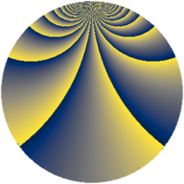# Properties

 Label 378.2.zLevel $378$ Weight $2$ Character orbit 378.z Rep. character $\chi_{378}(41,\cdot)$ Character field $\Q(\zeta_{18})$ Dimension $144$ Newform subspaces $1$ Sturm bound $144$ Trace bound $0$

# Related objects

## Defining parameters

 Level: $$N$$ $$=$$ $$378 = 2 \cdot 3^{3} \cdot 7$$ Weight: $$k$$ $$=$$ $$2$$ Character orbit: $$[\chi]$$ $$=$$ 378.z (of order $$18$$ and degree $$6$$) Character conductor: $$\operatorname{cond}(\chi)$$ $$=$$ $$189$$ Character field: $$\Q(\zeta_{18})$$ Newform subspaces: $$1$$ Sturm bound: $$144$$ Trace bound: $$0$$

## Dimensions

The following table gives the dimensions of various subspaces of $$M_{2}(378, [\chi])$$.

Total New Old
Modular forms 456 144 312
Cusp forms 408 144 264
Eisenstein series 48 0 48

## Trace form

 $$144 q - 12 q^{9} + O(q^{10})$$ $$144 q - 12 q^{9} + 12 q^{11} + 6 q^{14} + 12 q^{15} + 24 q^{21} - 60 q^{23} + 12 q^{29} - 72 q^{30} + 54 q^{35} - 12 q^{36} + 48 q^{39} + 24 q^{42} + 18 q^{49} - 60 q^{50} - 36 q^{51} + 6 q^{56} - 60 q^{57} - 36 q^{60} - 78 q^{63} + 72 q^{64} - 120 q^{65} + 36 q^{70} - 72 q^{71} - 24 q^{72} - 36 q^{74} + 66 q^{77} - 60 q^{78} - 72 q^{79} + 12 q^{84} - 72 q^{85} - 48 q^{86} - 18 q^{91} + 12 q^{92} + 24 q^{93} - 120 q^{95} + 36 q^{98} + 144 q^{99} + O(q^{100})$$

## Decomposition of $$S_{2}^{\mathrm{new}}(378, [\chi])$$ into newform subspaces

Label Dim $A$ Field CM Traces $q$-expansion
$a_{2}$ $a_{3}$ $a_{5}$ $a_{7}$
378.2.z.a $144$ $3.018$ None $$0$$ $$0$$ $$0$$ $$0$$

## Decomposition of $$S_{2}^{\mathrm{old}}(378, [\chi])$$ into lower level spaces

$$S_{2}^{\mathrm{old}}(378, [\chi]) \cong$$ $$S_{2}^{\mathrm{new}}(189, [\chi])$$$$^{\oplus 2}$$